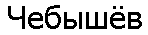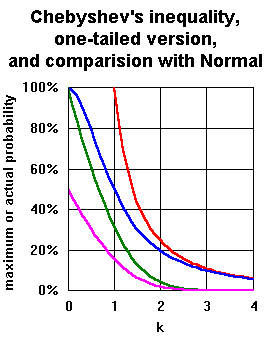## Chebyshev's inequality and a one-tailed version

 Chebyshev's inequality states that for t>0 P[|X-E(X)|>=t] <= Var(X)/t2 which is equivalent to for k>0 P[|X-E(X)|>=k.sd(X)] <= 1/k2 A one-tailed version of Chebyshev's inequality is that for t>0 P[X-E(X)>=t] <= 1/(1 + t2/Var(X)) i.e. P[X-E(X)>=t] <= Var(X)/(Var(X) + t2) which is equivalent to for k>0 P[X-E(X)>=k.sd(X)] <= 1/(1+k2) Turning inequality into equality Turning inequality into equality Proof of Chebyshev's inequality Proof of one-tailed version of Chebyshev's inequality Chebyshev or Tchebycheff? Speculation on unimodal PDFs

### Turning Chebyshev's inequality into an equality

<= becomes = when distribution X is (for given k>=1 and any m and any s>0)
P[X=m-k.s] = 1/(2.k2), P[X=m+k.s] = 1/(2.k2), and P[X=m] = 1-1/k2 .
Note E(X)=m and Var (X)=s2, sd(X)=s, so for this X  P[|X-E(X)|>=k.sd(X)] = 1/k2 .
The equality will in general only be achieved for a symmetric three-valued distribution. If the probabilities are p, 1-2p and p then equality is achieved when k=(2p)-1/2. A symmetric two-valued distribution is a special case with k=1.
A chart showing this distribution for k=2 is below.
Note also that for 1>k>0, 1/k2>1 which is not a probability, so achieving equality would be impossible.

### Turning one-tailed version of Chebyshev's inequality into an equality

<= becomes = when distribution X is (for given k>0 and any m and any s>0)
P[X=m+s.k] = 1/(1+k2), P[X=m-s/k] = k2/(1+k2) .
Note E(X)=m and Var (X)=s2, sd(X)=s, so for this X  P[X-E(X)>=k.sd(X)] = 1/(1+k2) .
The equality will in general only be achieved for a two-valued distribution. If the probability of the higher value is p (and the lower 1-p) then equality is achieved when k=(1/p-1)1/2
A chart showing this distribution for k=2 is below.
Note also that for all k>0, 1>1/(1+k2)>0 so, unlike the two-tailed version, this is always meaningful.

### Proof of Chebyshev's inequality

Markov's inquality states that for Y non-negative and s>0  P[Y>=s] <= E(Y)/s .
If Y=(|X-E(X)|)2 and s=t2 with t>0 then P[(|X-E(X)|)2>=t2] <= E(|X-E(X)|)2/t2
so P[|X-E(X)|>=t] <= Var(X)/t2 .
If t2=k2.Var(X) for k>0 then k.sd(X)=t and P[|X-E(X)|>=k.sd(X)] <= 1/k2 .

### Proof of one-tailed version of Chebyshev's inequality

If P[X-E(X)>=t] = 0 for given t>0 then clearly true,
otherwise let a = P[X-E(X)>=t], noting 0<a<1, and b = E(X-E(X)|X-E(X)>=t), noting t<=b,
and c = E((X-E(X))2|X-E(X)>=t), noting t2<=c<Var(X)/a

Consider T=t if X-E(X)>=t and T=0 if X-E(X)<t and
consider S=0 if X-E(X)>=t and S=(X-E(X)).t/b if X-E(X)<t :

then E(T) = a.t  and  E(S) = -a.b.t/b = -a.t  and  E(S|X-E(X)<t) = -a.t/(1-a)  and
E(T2) = a.t2 <= a.c  and  E(S2) = (Var(X)-a.c).t2/b2 <= Var(X)-a.c ,
so Var(X) >= E(T2)+E(S2) ;

but E(S2) = (1-a).E(S2|X-E(X)<t) >= (1-a).(E(S|X-E(X)<t))2=a2.t2/(1-a)
so Var(X ) >= a.t2+a2.t2/(1-a) = a.t2/(1-a) , so a <= 1/(1+t2/Var(X))

so P[X-E(X)>=t] <= 1/(1+t2/Var(X)) i.e. P[X-E(X)>=t] <= Var(X)/(Var(X)+t2) .
If t2=k2.Var(X) for k>0 then k.sd(X)=t and P[X-E(X)>=k.sd(X)] <= 1/(1+k2) .

### Alternative proofs of one-tailed version

The proof above was mine. A.G.McDowell has two at his page on Chebyshev's Inequalities: one of his own and one from 'Probability and Random Processes', by Grimmett and Stirzaker, published by Oxford Science Publications, ISBN 0 19 853665 8. The latter has the advantage of being short and (slightly adapted) is something like this:

With t>0, for any c>=0 P[X-E(X)>=t] = P[X-E(X)+c>=t+c] <= E((X-E(X)+c)2/(t+c)2) = (Var(X)+c2)/(t+c)2.
But treating (Var(X)+c2)/(t+c)2 as a function of c, the minimum occurs at c = Var(X)/t,
so P[X-E(X)>=t] <= (Var(X)+Var(X)2/t2)/(t+Var(x)/t)2 = Var(X)/(t2 + Var(x)).

In 2001 I produced yet another proof.

### Chebyshev or Tchebycheff

Pafnuty Lvovich Chebyshev was a notable Russian mathematician, who was born on 16 May 1821 and died on 8 December 1894. He wrote on number theory, analysis, probability, mechanics and maps.Being Russian, his name was written in the Cyrillic alphabet, causing problems of translitteration. Stijn van Dongen has compiled a list of 33 western European verions of his surname, of which Chebyshev seems to be the most popular in English, Tchebycheff in French, Cebysev in Spanish, and Tchebyscheff in German. Despited this variety, the final vowel always seems to be transcribed as "e" in that list when it is in fact pronounced "yo" in Russian. Even his given name and his patronimic have varying versions in roman script.

### Discussion

The two inequalities are very similar in form. However, for small k, they produce very different results. Indeed the one-tailed version produces meaningful results for 0<k<1, while Chebyshev's inequality less helpfully limits the probability to being less than or equal to a number greater than 1. For k=1, the one-tailed version provides the result that the median of a distribution is within one standard deviation of the mean. For large k, as shown in the table and chart below, the mamimum probability values given by the two inequalities are very similar. For large k, they are very different to the results for the Normal distribution.

 P(|X-E(X)|>=k.sd(X)) or P(X-E(X)>=k.sd(X))k Chebyshev's inequality One tailed version Normal two-tailed Normal one-tailed 0.1 (10000%) 99% 92.0% 46.0% 0.5 (400%) 80% 61.7% 30.9% 0.9 (123%) 55% 36.8% 18.4% 1 100% 50% 31.7% 15.9% 1.64 37.0% 27.0% 10% 5% 1.96 26.0% 20.7% 5% 2.5% 2 25% 20% 4.55% 2.28% 3 11.11% 10% 0.27% 0.13% 4 6.25% 5.88% 0.006% 0.003% 4.36 5.26% 5% 0.0013% 0.0007% 4.47 5% 4.76% 0.0008% 0.0004% 9.95 1.01% 1% close to 0 close to 0 10 1% 0.99% close to 0 close to 0

The similarity of the probability values for high k between Chebyshev's inequality and the one-tailed version is slightly misleading, as can be seen when the distribributions which turn the inequalities into equalities are considered, with examples in the chart below. The equality distribution for Chebychev's inequality is symmetric, while the equality distribution for the one-tailed version is not. For large k, the highest element of the former has only just over half the probability of the highest element of the latter.

Commenting in sci.stat.math, Herman Rubin, Department of Statisitics, Purdue University, said:

"This goes back more than a century. I do not know the history.

"This is the simplest of a class of inequalities, which I believe are due to Markov, a student of Chebyshev. A good book on the moment problem should have a reference.
If 1=m_0, m_1, ..., m_{2n} are the first 2n moments of a probability distribution,
let f be the unique (apart from a constant of proportionality) polynomial of degree at most n+1 with x as a root, and orthogonal to all polynomials of degree less than n.
If x is not a root of the orthogonal polynomial of degree n, there are n+1 roots, and the unique distribution concentrated at these roots fitting the given moments maximizes P(X <= x) and minimizes P(X < x).
If x is a root of the orthogonal polynomial, the 2n-th moment may not be matched at the n roots.

"In the case n=1, If x=E(X), the extreme distribution is concentrated at E(X).
Otherwise, if x = E(X)+t, the distribution is concentrated at x and E(X)-Var(X)/t."

### Some extra thoughts on Chebyshev type inequalities for unimodal distributions (October 1999):

If X is a continuous random variable with a unimodal probability density function (pdf), we may be able to tighten Chebyshev's inequality, though only by adding some complexity.

If the unimodal probability density function is also symmetric, then result
P[|X-E(X)|>=t] <= 4.Var(X)/(9.t2) or P[|X-E(X)|>=k.sd(X)] <= 4/(9.k2) applies for all t,k>0
This is Gauss's inquality of 1821 when the mode and mean are identical,
and is four ninths of the bound of Chebshev's inequality.
When 0<t2<=4.Var(X)/3 or 0<k<=2/sqrt(3) about 1.15470... I think this can be improved to
P[|X-E(X)|>=t] <= 1-t/sqrt(3.Var(X))
or P[|X-E(X)|>=k.sd(X)] <= 1-k/sqrt(3)
It is obvious that for a symmetric pdf, the one-sided bounds are half these.

If the unimodal pdf need not be symmetric then I suspect the inequality only applies for high t and k:
when t2>=B2.Var(X) or k>=B
where B is the largest root of 7.x6-14.x4-x2+4=0, about 1.38539...
P[|X-E(X)|>=t] <= 4.Var(X)/(9.t
2) or P[|X-E(X)|>=k.sd(X)] <= 4/(9.k2) as before,
but when 0<t2<=B2.Var(X) or 0<k<=B and with B as before, about 1.38539...
P[|X-E(X)|>=t] <= 1-(4.t
2/(3.(Var(X)+t2)))2 or P[|X-E(X)|>=k.sd(X)] <= 1-(4.k2/(3.(1+k2)))2
(I produced a sketch of why this is true in 2002)

The one sided version might be:
when t2>=5.Var(X)/3 or k>=sqrt(5/3), about 1.29099...
P[X-E(X)>=t] <= 4.Var(X)/(9.(Var(X)+t2)) or P[X-E(X)>=k.sd(X)] <= 4/(9.(1+k2))
this time four ninths of the one sided version of Chebyshev's inequality
and when 0<t2<=5.Var(X)/3 or 0<k<=sqrt(5/3)
P[X-E(X)>=t] <= 1-4.t
2/(3.(Var(X)+t2)) or P[X-E(X)>=k.sd(X)] <= 1-4.k2/(3.(1+k2))
(I have attempted a proof of this in 2001)

In an e-mail in October 2000, Michael Dummer commented:
"there is a simple unimodal distribution which meets the tighter Unimodal Chebyshev bound: a rectangular or uniform distribution with an impulse at the midpoint of the uniform range."

I responded:
"I think this applies to the symmetric unimodal case; for the non-symmetric unimodal two and one-sided cases, the bound is met for uniform distributions with an impulse away from the midpoint." Perhaps I should have added the bounds for small t and k are achieved for a uniform distribution with an impulse at one end where the probability of the uniform distribution is 4.k2/(3.(1+k2)).

I have developed a page which takes the one-tailed case a little further (especially the unimodal case) as well as some median-mean-mode inequalities.

E-mail me your thoughts